Clutch Prep is now a part of Pearson
Ch.6 - Chemical EquilibriumWorksheetSee all chapters

# Le Chatelier's Principle

See all sections
Sections
The Equilibrium State
The Reaction Quotient
Le Chatelier's Principle
Chemical Thermodynamics: Enthalpy
Chemical Thermodynamics: Entropy
Chemical Thermodynamics: Gibbs Free Energy
Solubilty Product Constant
Protic Acids and Bases
The pH Scale
Acid Strength

Le Chatelier's Principle discusses the shifting pattern of a chemical reaction in order to maintain an equilibrium state.

###### Chemical Thermodynamics

Concept #1: The addition or removal of products or reactants causes the chemical reaction to shift in the direction necessary to re-establish equilibrium.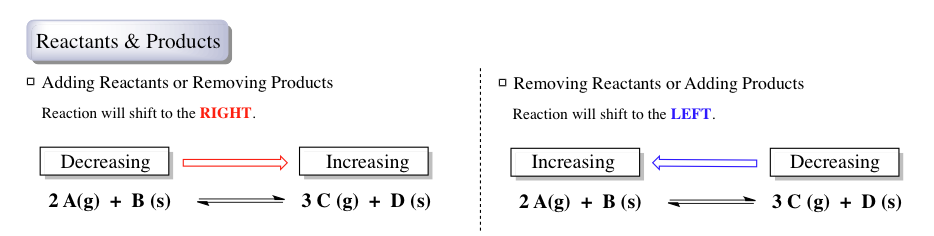Concept #2: Increasing or decreasing pressure or volume causes the reaction to shift in the direction necessary to re-establish equilibrium.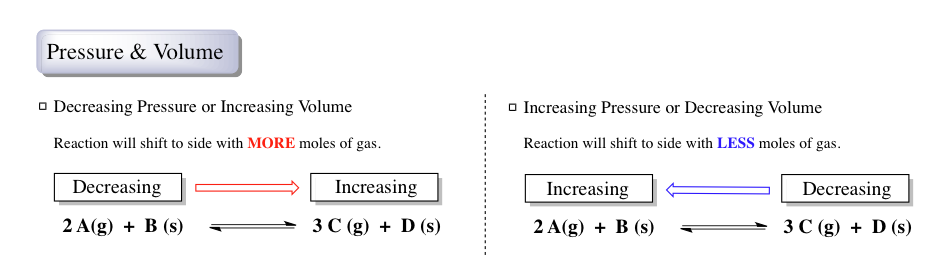Concept #3: Increasing or decreasing temperature causes the reaction to shift in the direction necessary to re-establish equilibrium.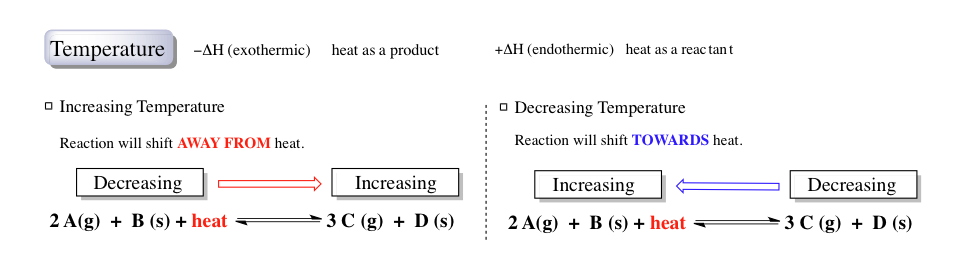Concept #4: Depending on the conditions, the addition or removal of a noble gas can potentially cause the reaction to shift in the direction necessary to re-establish equilibrium.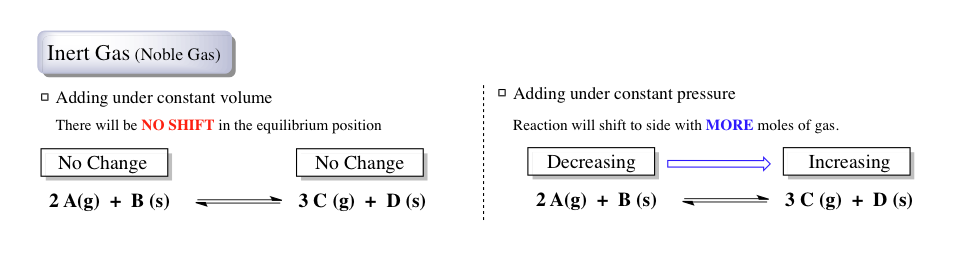Under equilibrium conditions, the addition or removal of a liquid, solid or catalyst will cause no shift in the reaction's position.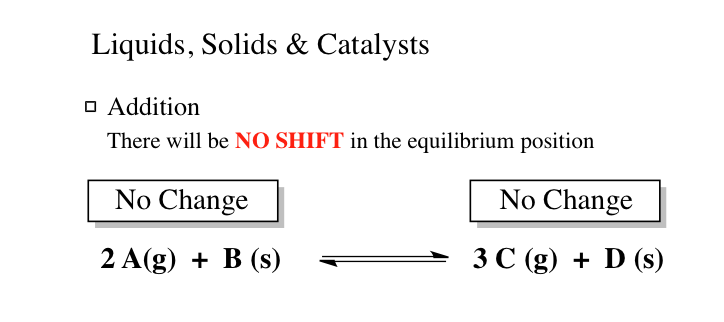Example #1: The following data was collected for the following reaction at equilibrium.

A (s)  + 2 B  (g) ⇌ 3 C (g) +  D (g)

At 55oC, K is 4.7 x 10-7 and at 100oC K is 1.9 x 10-2. Which of the following statements is true?

a) The reaction is exothermic.

b) The reaction is endothermic.

c) The enthalpy change, ΔH, is equal to zero.

d) Not enough information is given

Example #2: In which of these gas-phase equilibria is the yield of products increased by increasing the total pressure on the reaction mixture?

a) CO (g) +  H2O (g) ⇌  CO2 (g)  +  H2 (g)

b) 2 NO (g)  +  Cl2 (g) ⇌  2 NOCl (g)

c) 2 SO3 (g) ⇌  2 SO2 (g)  +  O2 (g)

d) PCl5 (g) ⇌  PCl3 (g)  +  Cl2 (g)

e) 2 H2O2 (g) ⇌ 2 H2O (g)  +  O2 (g)

Practice: The reaction 2 NaHCO(s) ⇌ Na2CO(s) + H2O (g) + CO(g) is endothermic. What would you do in order to maximize the yield of Na2CO(s)?